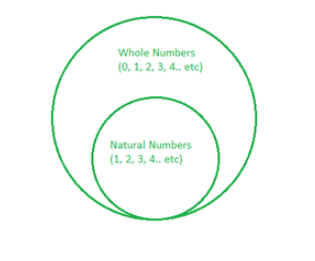# What are Whole Numbers? – Definition, Properties, Examples

• Last Updated : 05 Aug, 2021

The way of representing the numbers using digits, symbols are called the number system. It is also called the set of values that are used to represent quantity and things. There are different types of numbers based on the distinct features of the numbers, for instance, all numbers that are divisible by 2 are Even numbers, the number that is only divisible by 1 or itself is a Prime number. Numbers generating from 1 and going up to infinity are Natural numbers while the numbers generating from 0 and going up to infinity are whole numbers, Let’s take a closure look at what are whole numbers,

### What are whole numbers?

The natural number starting with 0 is called a whole number. The numbers 0, 1, 2, 3, 4, 5, 6, and (so on) are the whole numbers. It is the set of positive integers. It can be said that The whole number is the set of numbers without fractions, decimals, and negative numbers. It is set of only positive numbers including 0. The number smallest among the whole numbers is 0. All whole numbers are also real numbers. Every natural number is a Whole number but not vice-versa.

Attention reader! All those who say programming isn't for kids, just haven't met the right mentors yet. Join the  Demo Class for First Step to Coding Course, specifically designed for students of class 8 to 12.

The students will get to learn more about the world of programming in these free classes which will definitely help them in making a wise career choice in the future.Relation between Whole numbers and Natural numbers

Properties of Whole numbers

•  Closure Property

In this property, if x+y be the whole number then y+x also be the whole number.

In this property, the sum of any two whole numbers is the same. i.e., the order of addition doesn’t matter. i.e.,

x+y = y+x.

• Commutative Property of Multiplication

In this property, the multiplication of any two whole numbers is the same. i.e., any number can be multiplied in any order. i.e.,    x× y = y× x.

In this property, When we add the value with zero then the value of the integer remains unchanged. i.e.,

x+0 = 0+x.

•  Multiplicative Identity

In this property, When we multiply the value with 1 then the value of the integer remains unchanged. i.e.,

x× 1 = 1× x.

•  Associative Property

In this property, When add and multiply the number and grouped together in any order the value of the resultant remains same. i.e.,

x+( y+ z) = (x+ y)+ z and x× (y× z) = (x×y)× z

•  Distributive Property

In this property, When multiply the number and distributed them in any order the value of the resultant remains same. i.e.,

x×(y+z) =(x× y) + (x× z).

Question 1: Are the numbers 100, 399, 457 are the whole numbers?

Yes, the numbers 100, 399, 457 are the whole numbers.

Question 2: Solve the equation 15 × (10 +5) using the distributive property.